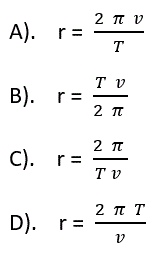NEED HELP? Edexcel iGCSE digital textbooks From the makers of Grade Gorilla. GGebooks.com1. Mars has a smaller gravitational field strength at the surface than the Earth. This is because...

• A) the mass of Mars is smaller than Earth.
• B) Mars has a very thin atmosphere.
• C) Mars has no water on the surface.
• D) Mars orbits the Sun at a slower speed than Earth.2. Which of the following answers best describes the orbits of the Earth, the (Earth's) Moon and a comet?

 Near circular orbit Highly elliptical (non-circular) orbit A. Earth Moon, a comet B. Earth, Moon a comet C. a comet, Moon Earth D. Moon a comet, Earth

3. Which of the following gives the sizes of astronomical objects in order of increasing size?

• A) Solar system, universe, galaxy.
• B) Universe, galaxy, solar system.
• C) Solar system, galaxy, universe.
• D) Galaxy, solar system, universe.

4. Approximately how many stars are in a typical galaxy?

• A) Hundreds of stars.
• B) thousands of stars.
• C) millions of stars.
• D) billions of stars.

5. What is the name of our own galaxy containing the Sun?

• A) The Andromeda galaxy.
• B) The Milky Way galaxy.
• C) The Orion galaxy.
• D) The Hubble galaxy.

6. Which of these statements is correct?

• A). Planets orbit moons, planets orbit stars.
• B). Moons orbit planets, stars orbit planets.
• C). Moons orbit stars, stars orbit planets.
• D). Moons orbit planets, planets orbit stars.7. The formula on the right can be used to calculate the orbital velocity v of any object. It can be re-arranged to find the radius of the orbit.Which of the following has been correctly re-aranged?8. The radius of the Earth's orbit is about 150 million kilometres. In standard form, and given in metres, this is ..

• A). 1.5 x 108 m
• B). 1.5 x 1010 m
• C). 1.5 x 1011 m
• D). 1.5 x 1012 m

9. The Earth orbits the Sun in 1 year, which is 3.2 x 107 seconds. Using this figure, along with information given in questions 7 and 8, what is the orbital velocity of the Earth?

• A). 4.6 x 103 m/s
• B). 29 m/s 103
• C). 4.6 m/s 103
• D). 2.9 x 104 m/s